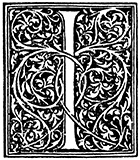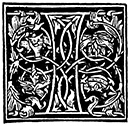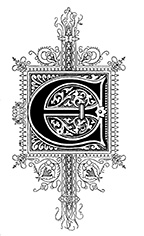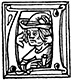﻿ Newton's First Law | Zona Land Education

# Ways To Say Newton's First Law

Here is a collection of statements of Isaac Newton's first law of motion in words similar to those often presented in high school and college textbooks. Click the 'More' button near each statement, and the page will expand with an explanation.

The central idea of the First Law is that forces are necessary to change the velocity of an object. An object will not spontaneously change its velocity unless it is pushed by an external force. Remember that a change in velocity would mean that the object speeds up, slows down, or travels in a curved path.f there is no net force acting on a body, it will continue in its state of rest or will continue moving along in a straight line with uniform velocity.

 'If there is no net force acting on a body' The net, or total, force acting on the object is zero Newtons. This means one of two things. There could be no forces acting on the object. Or there could be several forces acting on the object, but they push against each other and cancel, and the result is as if no force is acting. 'it will continue in its state of rest' If it is standing still, it will continue to stand still. This means that if the velocity is zero, the velocity will continue to be zero. Also, this states that the velocity will remain constant; it will constantly remain zero. Finally, this means that if the object is still, it will not start moving all on its own with no push or pull from the outside. "continue moving along in a straight line with uniform velocity" The velocity of the object will remain constant. It will move in a straight line. It will not change direction. It will not follow a curved path. Uniform velocity means constant velocity. Velocity is a vector, so it has size and direction. Constant velocity means moving at constant speed (velocity's size) in a straight line (velocity's direction). Note that to say 'uniform velocity' already means moving in a straight line. Perhaps this last part could be stated 'continue moving along in a straight line with uniform speed'. That would remove the small redundancy.n object at rest remains at rest if there is zero resultant force acting on it. If the resultant force acting on a moving object is zero, the object continues its motion with constant velocity.

 'An object at rest will remain at rest' If the velocity is zero, it will remain zero. Note this means the velocity is remaining constant. Also note this means if the object is standing still, it will continue to stand still - it will not start moving on its own without a push. 'zero resultant force' The net or total force is zero. Either there are absolutely no forces acting on the object, or there are several, but when you add them all together, they cancel each other out. 'continues its motion with constant velocity' Constant velocity means motion in a straight line with unchanging speed.f the vector sum of the external forces acting on an object is zero, the velocity of the object remains constant.

 'the vector sum of the external forces acting on an object is zero' There may be several forces acting on the object, but when they are added together, the result is that they cancel each other out. This makes the net force zero. 'the velocity of the object remains constant' The object's motion is in a straight line at constant speed. Or if it is standing still with a velocity of zero, then it will continue to have that velocity of zero, it will continue to stand still.very body continues in its state of rest or of uniform speed in a straight line unless acted on by a non-zero net force.

 'continues in its state of rest' If the object's velocity is zero, then its velocity will remain zero. 'continues in its state ... of uniform speed in a straight line' Constant velocity is uniform speed in a straight line. So this means the object will continue at a constant velocity. 'unless acted on by a non-zero net force' The velocity will not remain constant if the net force is not zero. In other words, if there is any amount (can not be zero amount) of total, or net, force acting on the object, the object's velocity will change.n object at rest remains at rest, and an object in motion continues in motion with constant velocity unless it experiences a net external force.

 'An object at rest remains at rest' If the velocity of the object is zero, it will continue to be zero. This means the velocity stays constant with a value of zero. This means the object will not start to move spontaneously, without a push. 'an object in motion continues in motion with constant velocity. If it is moving, it will continue to move at a constant speed in a straight line. 'unless it experiences a net external force' The velocity will not be constant, that is, it will change, if there is an actual net force on the object that is not a zero net force.nless acted on by an outside force, an object at rest, stays at rest; and unless acted on by an outside force, an object moving with uniform velocity continues to move at that velocity.

 'Unless acted on by an outside force' This means unless the net force on the object is not zero. Here, this could be translated 'When the net force is zero'. 'an object at rest, stays at rest' If the velocity is zero, it will stay zero. Note this means the velocity will remain zero constantly. It also means the object will not start moving unless you push on it. 'an object moving with uniform velocity continues to move at that velocity' If the object is moving at constant speed in a straight line, it will continue to move at constant speed in a straight line.very body continues in its state of rest or uniform motion in a straight line unless it is compelled to change that state by forces impressed upon it.

 'continues in its state of rest' If the object's velocity is zero, it will continue to be zero. In other words, its velocity will stay constantly zero, and it will not start to move on its own without a push from the outside. 'uniform motion in a straight line' 'Uniform motion' translates to 'constant speed' here. So this reads 'constant speed in a straight line.' Constant speed in a straight line is constant velocity. 'compelled to change that state by forces impressed upon it' The velocity will change if the object experiences a non-zero net, or total, force.body continues in a state of rest or uniform motion in a straight line unless it is acted upon by external forces.

 'continues in a state of rest' If the object's velocity is zero, it will continue to constantly be zero. It will not start to move on its own without an external push. 'uniform motion in a straight line' This means constant speed in a straight line, and that means constant velocity. So if the object has a constant velocity, it will keep that constant velocity. 'unless acted upon by external forces' The velocity will not stay constant if there is a net force on the object larger than zero.

Custom Search